# RD Sharma Solutions for Class 9 Maths Chapter 5 Factorization of Algebraic Expressions Exercise 5.1

RD Sharma Class 9 Mathematics Chapter 5 Exercise 5.1 Factorization of Algebraic Expressions is provided here. In this chapter, we will solve different types of algebraic expressions like monomials, binomials, trinomials, and polynomials using identities. Understand these concepts and have in-depth knowledge through some of the solved RD Sharma solutions for 9th standard. BYJU’S experts have prepared RD Sharma Solutions Class 9 chapter 5 as per latest CBSE syllabus for class 9. Students can download RD Sharma exercise 5.1 by clicking on the link below.

## Download PDF of RD Sharma Solutions for Class 9 Maths Chapter 5 Factorization of Algebraic Expressions Exercise 5.1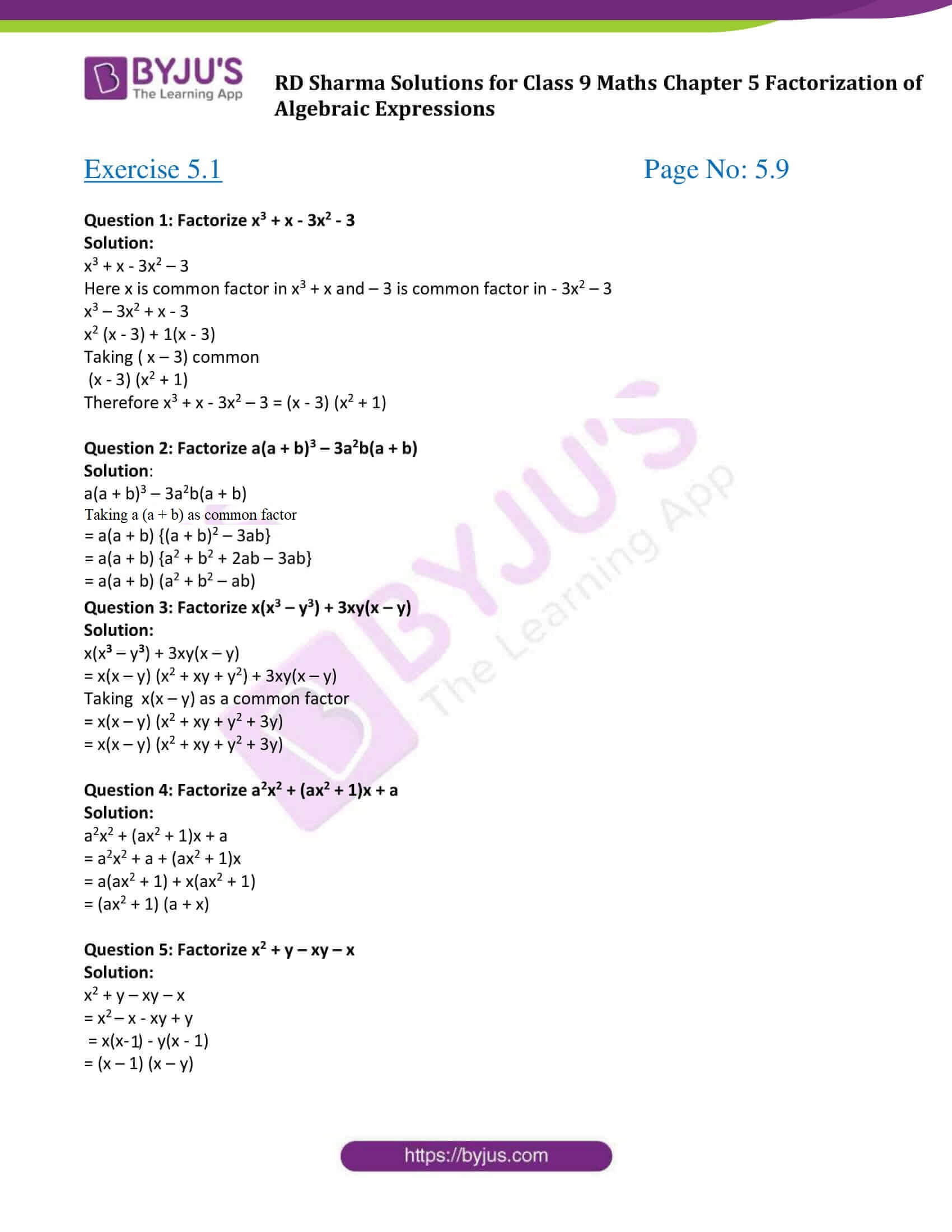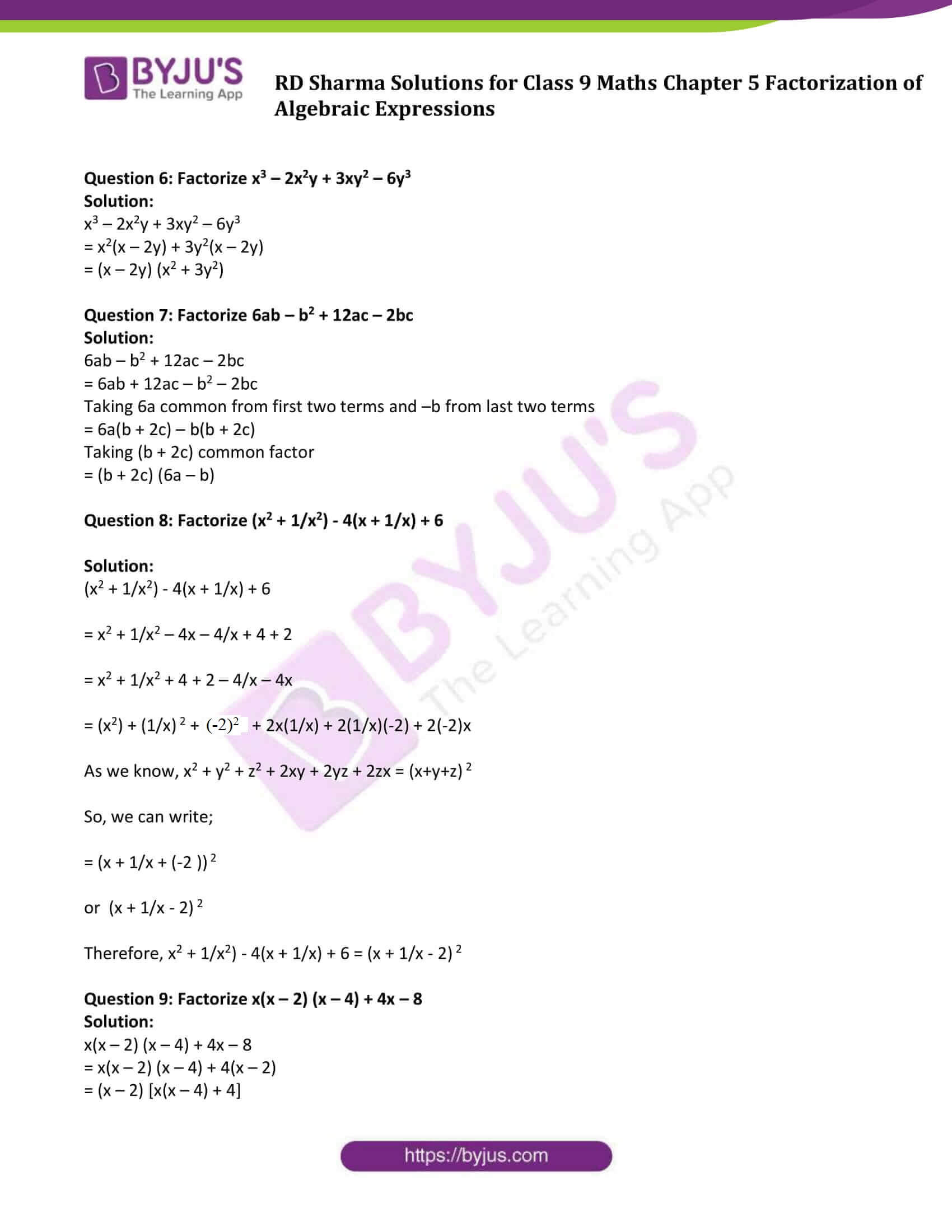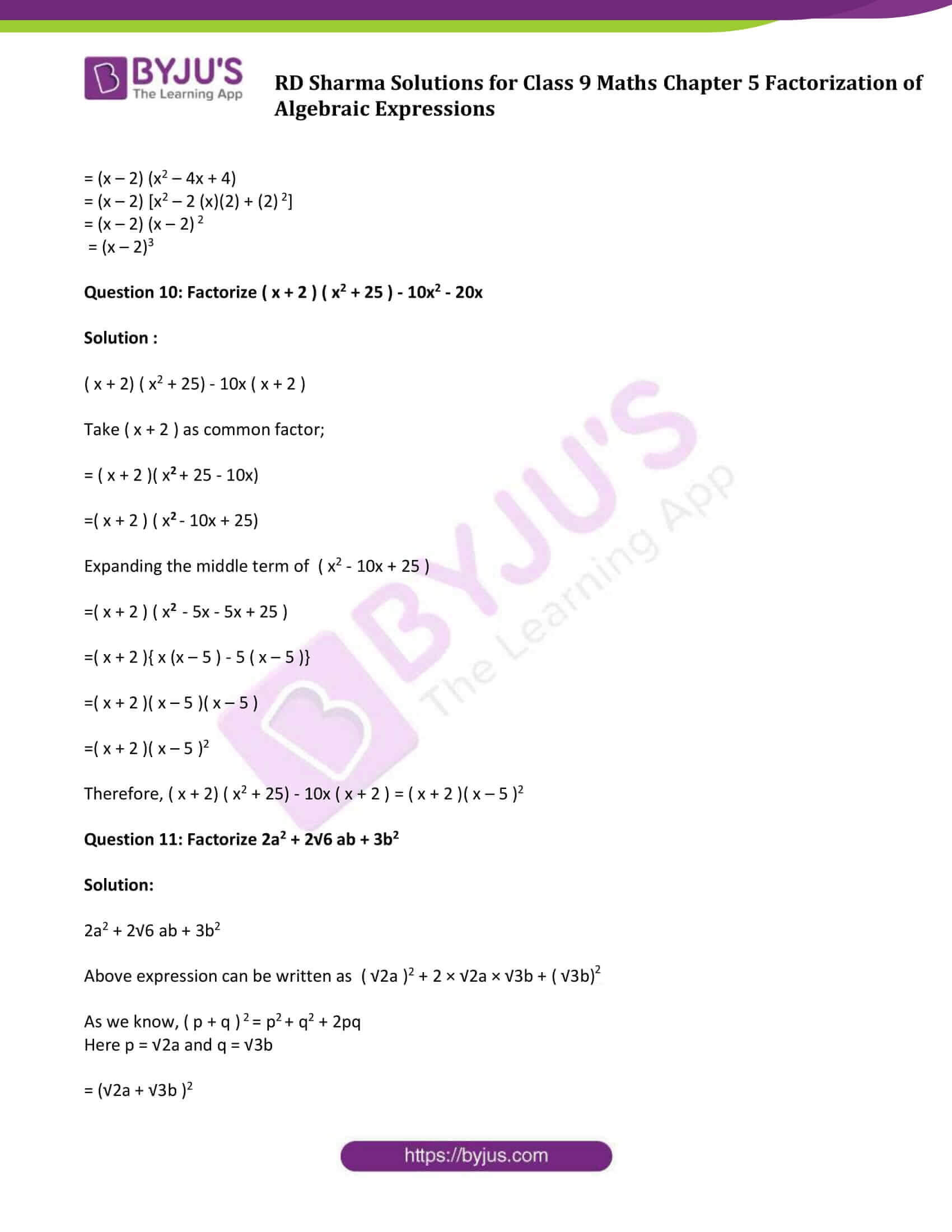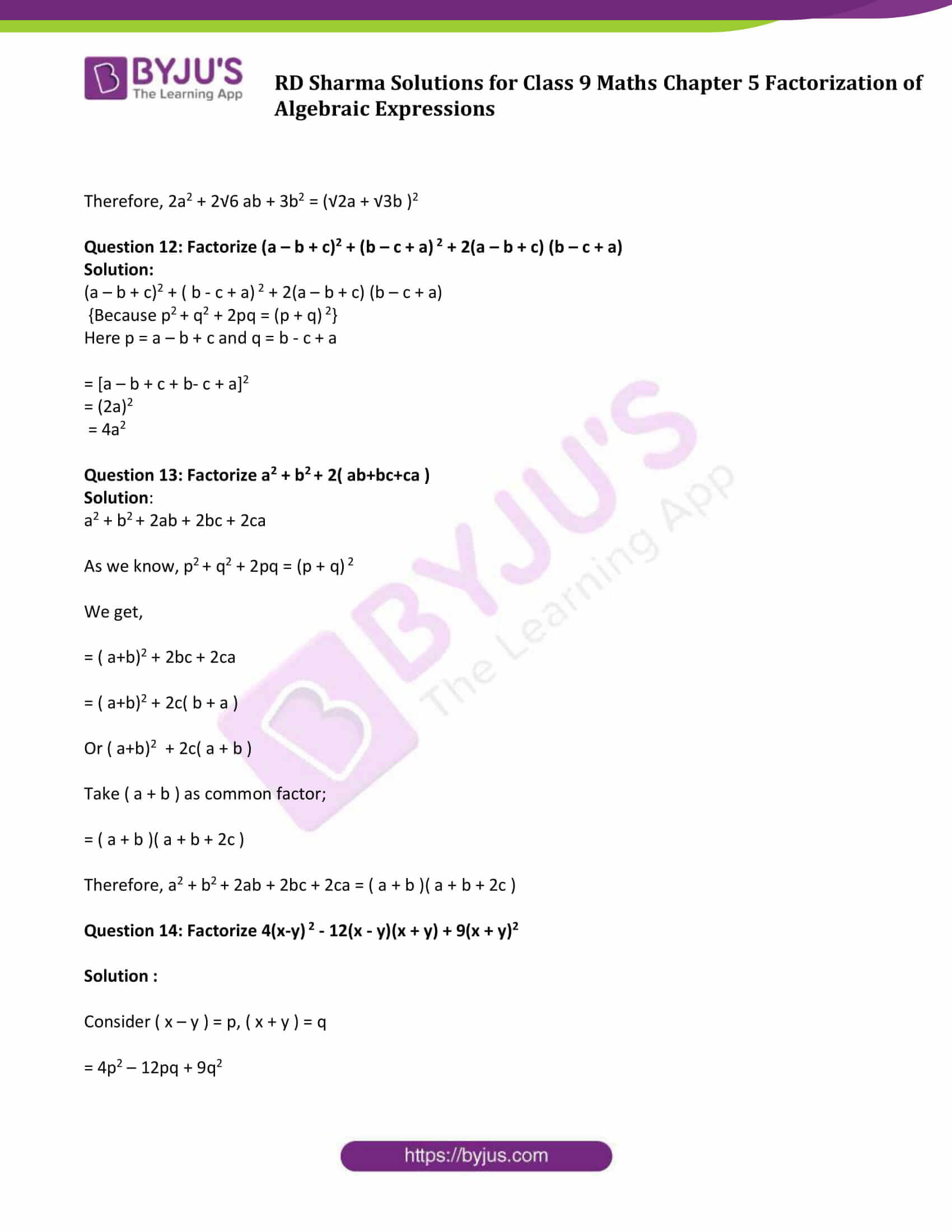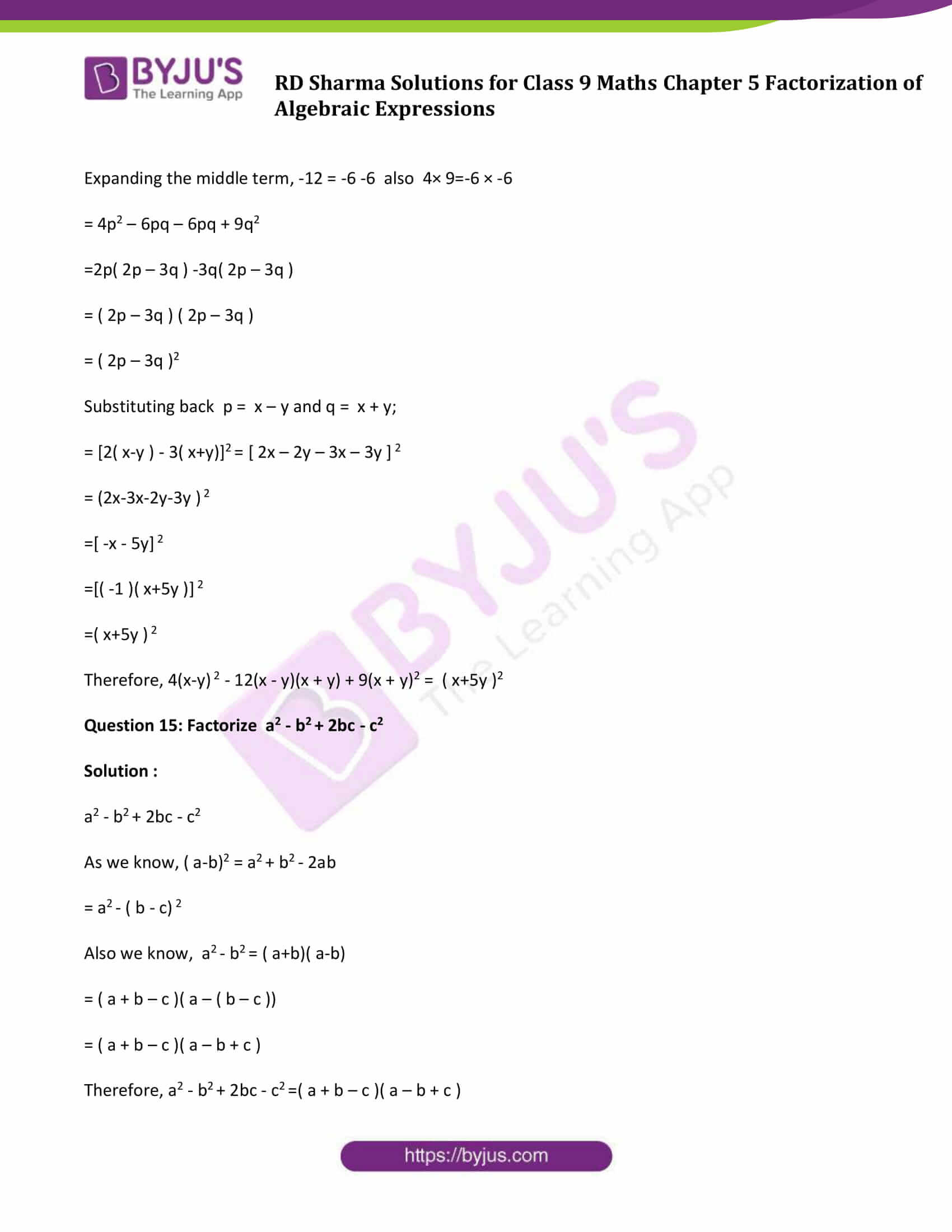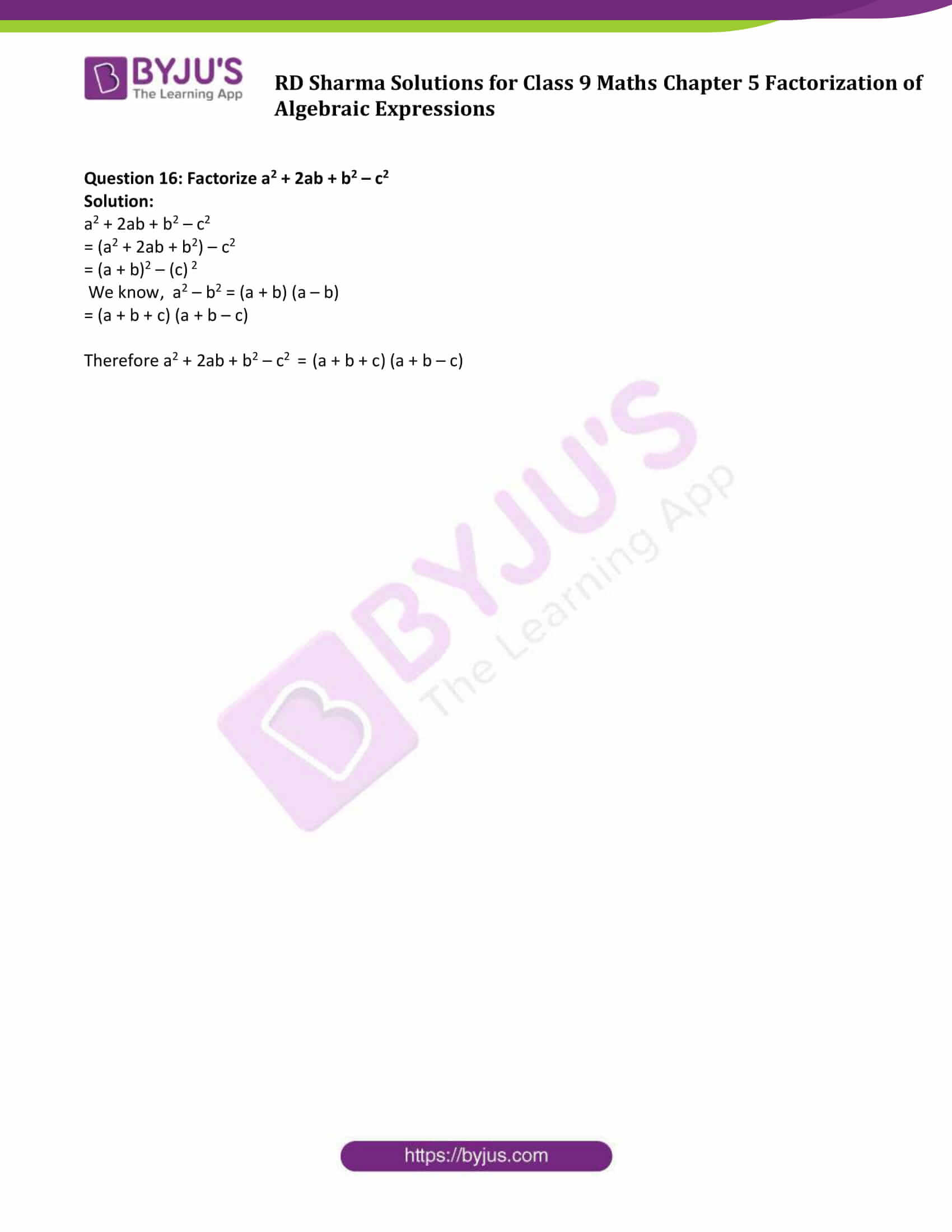### Access Answers to Maths RD Sharma Solutions for Class 9 Chapter 5 Factorization of Algebraic Expressions Exercise 5.1 Page number 5.9

#### Exercise 5.1 Page No: 5.9

Question 1: Factorize x3 + x – 3x2 – 3

Solution:

x3 + x – 3x2 – 3

Here x is common factor in x3 + x and – 3 is common factor in – 3x2 – 3

x3 – 3x2 + x – 3

x2 (x – 3) + 1(x – 3)

Taking ( x – 3) common

(x – 3) (x2 + 1)

Therefore x3 + x – 3x2 – 3 = (x – 3) (x2 + 1)

Question 2: Factorize a(a + b)3 – 3a2b(a + b)

Solution:

a(a + b)3 – 3a2b(a + b)

Taking a(a + b) as common factor

= a(a + b) {(a + b)2 – 3ab}

= a(a + b) {a2 + b2 + 2ab – 3ab}

= a(a + b) (a2 + b2 – ab)

Question 3: Factorize x(x3 – y3) + 3xy(x – y)

Solution:

x(x3 – y3) + 3xy(x – y)

= x(x – y) (x2 + xy + y2) + 3xy(x – y)

Taking x(x – y) as a common factor

= x(x – y) (x2 + xy + y2 + 3y)

= x(x – y) (x2 + xy + y2 + 3y)

Question 4: Factorize a2x2 + (ax2 + 1)x + a

Solution:

a2x2 + (ax2 + 1)x + a

= a2x2 + a + (ax2 + 1)x

= a(ax2 + 1) + x(ax2 + 1)

= (ax2 + 1) (a + x)

Question 5: Factorize x2 + y – xy – x

Solution:

x2 + y – xy – x

= x2 – x – xy + y

= x(x- 1) – y(x – 1)

= (x – 1) (x – y)

Question 6: Factorize x3 – 2x2y + 3xy2 – 6y3

Solution:

x3 – 2x2y + 3xy2 – 6y3

= x2(x – 2y) + 3y2(x – 2y)

= (x – 2y) (x2 + 3y2)

Question 7: Factorize 6ab – b2 + 12ac – 2bc

Solution:

6ab – b2 + 12ac – 2bc

= 6ab + 12ac – b2 – 2bc

Taking 6a common from first two terms and –b from last two terms

= 6a(b + 2c) – b(b + 2c)

Taking (b + 2c) common factor

= (b + 2c) (6a – b)

Question 8: Factorize (x2 + 1/x2) – 4(x + 1/x) + 6

Solution:

(x2 + 1/x2) – 4(x + 1/x) + 6

= x2 + 1/x2 – 4x – 4/x + 4 + 2

= x2 + 1/x2 + 4 + 2 – 4/x – 4x

= (x2) + (1/x) 2 + (-2)2 + 2x(1/x) + 2(1/x)(-2) + 2(-2)x

As we know, x2 + y2 + z2 + 2xy + 2yz + 2zx = (x+y+z) 2

So, we can write;

= (x + 1/x + (-2 )) 2

or (x + 1/x – 2) 2

Therefore, x2 + 1/x2) – 4(x + 1/x) + 6 = (x + 1/x – 2) 2

Question 9: Factorize x(x – 2) (x – 4) + 4x – 8

Solution:

x(x – 2) (x – 4) + 4x – 8

= x(x – 2) (x – 4) + 4(x – 2)

= (x – 2) [x(x – 4) + 4]

= (x – 2) (x2 – 4x + 4)

= (x – 2) [x2 – 2 (x)(2) + (2) 2]

= (x – 2) (x – 2) 2

= (x – 2)3

Question 10: Factorize ( x + 2 ) ( x2 + 25 ) – 10x2 – 20x

Solution :

( x + 2) ( x2 + 25) – 10x ( x + 2 )

Take ( x + 2 ) as common factor;

= ( x + 2 )( x2 + 25 – 10x)

=( x + 2 ) ( x2 – 10x + 25)

Expanding the middle term of ( x2 – 10x + 25 )

=( x + 2 ) ( x2 – 5x – 5x + 25 )

=( x + 2 ){ x (x – 5 ) – 5 ( x – 5 )}

=( x + 2 )( x – 5 )( x – 5 )

=( x + 2 )( x – 5 )2

Therefore, ( x + 2) ( x2 + 25) – 10x ( x + 2 ) = ( x + 2 )( x – 5 )2

Question 11: Factorize 2a2 + 2√6 ab + 3b2

Solution:

2a2 + 2√6 ab + 3b2

Above expression can be written as ( √2a )2 + 2 × √2a × √3b + ( √3b)2

As we know, ( p + q ) 2 = p2 + q2 + 2pq

Here p = √2a and q = √3b

= (√2a + √3b )2

Therefore, 2a2 + 2√6 ab + 3b2 = (√2a + √3b )2

Question 12: Factorize (a – b + c)2 + (b – c + a) 2 + 2(a – b + c) (b – c + a)

Solution:

(a – b + c)2 + ( b – c + a) 2 + 2(a – b + c) (b – c + a)

{Because p2 + q2 + 2pq = (p + q) 2}

Here p = a – b + c and q = b – c + a

= [a – b + c + b- c + a]2

= (2a)2

= 4a2

Question 13: Factorize a2 + b2 + 2( ab+bc+ca )

Solution:

a2 + b2 + 2ab + 2bc + 2ca

As we know, p2 + q2 + 2pq = (p + q) 2

We get,

= ( a+b)2 + 2bc + 2ca

= ( a+b)2 + 2c( b + a )

Or ( a+b)2 + 2c( a + b )

Take ( a + b ) as common factor;

= ( a + b )( a + b + 2c )

Therefore, a2 + b2 + 2ab + 2bc + 2ca = ( a + b )( a + b + 2c )

Question 14: Factorize 4(x-y) 2 – 12(x – y)(x + y) + 9(x + y)2

Solution :

Consider ( x – y ) = p, ( x + y ) = q

= 4p2 – 12pq + 9q2

Expanding the middle term, -12 = -6 -6 also 4× 9=-6 × -6

= 4p2 – 6pq – 6pq + 9q2

=2p( 2p – 3q ) -3q( 2p – 3q )

= ( 2p – 3q ) ( 2p – 3q )

= ( 2p – 3q )2

Substituting back p = x – y and q = x + y;

= [2( x-y ) – 3( x+y)]2 = [ 2x – 2y – 3x – 3y ] 2

= (2x-3x-2y-3y ) 2

=[ -x – 5y] 2

=[( -1 )( x+5y )] 2

=( x+5y ) 2

Therefore, 4(x-y) 2 – 12(x – y)(x + y) + 9(x + y)2 = ( x+5y )2

Question 15: Factorize a2 – b2 + 2bc – c2

Solution :

a2 – b2 + 2bc – c2

As we know, ( a-b)2 = a2 + b2 – 2ab

= a2 – ( b – c) 2

Also we know, a2 – b2 = ( a+b)( a-b)

= ( a + b – c )( a – ( b – c ))

= ( a + b – c )( a – b + c )

Therefore, a2 – b2 + 2bc – c2 =( a + b – c )( a – b + c )

Question 16: Factorize a2 + 2ab + b2 – c2

Solution:

a2 + 2ab + b2 – c2

= (a2 + 2ab + b2) – c2

= (a + b)2 – (c) 2

We know, a2 – b2 = (a + b) (a – b)

= (a + b + c) (a + b – c)

Therefore a2 + 2ab + b2 – c2 = (a + b + c) (a + b – c)

## RD Sharma Solutions for Class 9 Maths Chapter 5 Factorization of Algebraic Expressions Exercise 5.1

RD Sharma Solutions Class 9 Maths Chapter 5 Factorization of Algebraic Expressions Exercise 5.1 is based on the following topics:

• Factorisation by taking out the common factors
• Factorisation by grouping the terms
• Factorisation by making a perfect square
• Factorisation by the difference of two squares
• Factorisation of quadratic polynomials by splitting the middle term